All submissions of the EM system will be redirected to Online Manuscript Submission System. Authors are requested to submit articles directly to Online Manuscript Submission System of respective journal.

# Research

, Volume: 7( 3) DOI: 10.37532/2320-6756.2019.7(3).180

## B FengÃ¢ÂÂs Theory (Part 5): Proof of 5-Dimensional Space

*Correspondence:
Mei ZH , College of Chemistry and Molecular Engineering, Qingdao University of Science and Technology, Qingdao, China, Tel: +86-0532-84022681; E-Mail: mzh62@qust.edu.cn

Received: May 11, 2019; Accepted: May 17, 2019; Published: May 23, 2019

Citation: Mei ZH. B Feng’s Theory (Part 5): Proof of 5-Dimensional Space. J Phys Astron. 2019;7(2):180.

### Abstract

According to B. Feng’s theory, the existence of 5-D space is proofed by its positive results which obtained. The evidences are the deduction of Lorentz transformation, the coherence with general relativity, the accurate calculation of electric charge, the accurate mass calculation of electron and proton, fine structure constant explanation and the establishing of unified field theory.

### Keywords

Five-dimensional Space; B Feng’s Theory; Kaluzer-Klein’s Theory; Elementary Particle Physics; 11-Dimensional Space

### Introduction

Karuza-Klein theory was abandoned by the mainstream of physics because of its incorrect result in the application of calculating the mass-charge ratio of the electron. However, we insist on and inherit Kaluza’s idea and published a series of theoretical papers [1-5] and application papers [6-10]; where a new physical theory was established and named by us as B. Feng’s. According to our B. Feng’s theory, we revealed the essence of the matter, especially the attribute of mass and charge of elementary particles, their accurate values were calculated; the top-level task of Unified Field Theory and other key problems were then accomplished or solved smoothly. In our theory, everything is attributed to vibration of vacuum (i.e. the second order vacuum in literature ). So, the property especially the dimensions of space is the first to be ascertained. In theory, the vacuum space is five-dimensional. The purpose of this paper is to confirm its correctness and make it a clear and intuitive understanding of common.

### Material and Methods

Supporting materials of 5-dimensional space

The description of classification can be as this: in physical change processes, the amount and types of molecules are unchangeable; in chemical reaction processes, the amount and types of atoms are unchangeable; in nuclear reaction processes, the amount and types of elementary particles are unchangeable; in elementary particle reaction processes, the amount and types of elementary particles is changeable, however, the charge is conservative.

This regularity of the classification inspires us the next deeper types of reaction, the charge reaction; in this type of reaction processes, the charges and the implicated rest mass are disappeared, nevertheless, the energy is conserved only.

From special relativity perspective

In special relativity, space (or motion) is relative. Lorentz discovered the relationship of Lorentz transformation in 1904 under the principle of constant light speed. On this basis, through performing a collision thought the experiment of two objects by using the conservation law of momentum, Einstein deduced the famous time-speed, mass-speed, and mass-energy, etc. formulas in 1905.

In H. Poincaré’s view in 1905, the effect of relativity is due to the existence of the fourth dimension (ict) in space. Imagining that, at a moment t, there is an observed target point “P” in the 4-dimensional space. Assuming an inertial reference system B(x, y, z)+T(ict) is moving relative to reference B=Ω(x, y, z)+T(ict) at velocity V in the 4-dimensional coordinate, let them coincide at the original point. Because of the principle of constant light speed, in 4-dimensional space, the relation Ω’2+T’22+T2 must be satisfied. So, it becomes obligatory that B’ and B are in rotational relations; the velocity, V, corresponds to the angle between the two coordinates (Fig. 1). Based on this, the results of Lorentz transformation can be easily deduced in another way. The process is as follows:

As shown in Fig. 1, we get(1)

Let Ω’=0, and Substituting in (1), one can have(2)

For simplifying, let Ω(x, y, z)=Ω(x, 0, 0), (1) and (2) become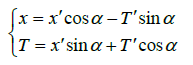(3)(4)

Solving equation (3) and (4), one can have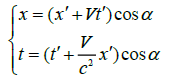(5)

As, we obtained(6)

Combining (5) and (6), the Lorentz transformation results. It must be noticed that where the angle α is a complex number. Above derivative is quite simple. That’s why we always say that the special relativity corresponds to 4-dimensional spacetime.

From general relativity perspective

In general relativity, Einstein’s field equations are expressed as, and in vacuum= 0

where, Gμv is Einstein tensor,the Ricci tensor, R the standard 4D Ricci scalar and R=gμv, Tμv the energy-momentum tensor, G the gravitational constant, c the speed of light, μ and v are indexes of coordinates t, x, y, z with 1, 2, 3, and 4, gμv the Minkowski space-time metric; because of the invariance of light, it has matrix form asThe following: indices a,b∈{1,2,3,4,5}, ø is expressed scalar field, Aμ and Av the electromagnetic potential vector,the metric tensor in five dimensions, the symbols with hat “~” mean those in five-dimensional.

Kaluza is the first to extend general relativity Einstein field equations to five dimensions: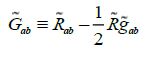, by hypothesis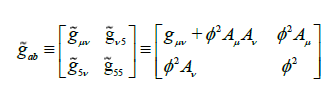The supposed metric form implies invariant five-dimensional length element ds: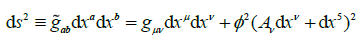The components of the Ricci tensor (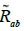) in Kaluza-Klein theory were detailed in [11-13], where coordinate (t, x, y, z,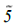) expressed with spherical one as (r, θ,φ , y).

The resulted full Kaluza equations are quite complex even under “cylinder condition”. The full equations are not shown here, however, can be evaluated using tensor algebra software in 2015.

The cylinder condition request that no component of the five-dimensional metric depends on the fifth dimension; that is=0

In order to explain the cylinder condition, 1926, O. Klein added a new hypothesis to the theory that the fifth dimension was compactified or curled up in a circle with the radius of 10−30 cm (the size of Plank length), who gave the Kaluza-Klein theory a quantum explanation; i.e., Klein’s an important contribution is in that who revealed the electric charge nature to be standing waves motion in the fifth dimension.

In a vacuum=0, means alsoAccordingly, Thiry obtained the electromagnetic potential vector Av fromIt has the form of the vacuum Maxwell equations if the scalar field ϕ is constant.

Defining an action S(g) asBy applying the variation principle to the action,, one obtains precisely the Einstein equations for free space:It is to say that the four-dimensional Einstein field equations and the Maxwell electromagnetic field equations can all be derived in five-dimensional space; it unified the electromagnetic field and gravity. This means that the five-dimensional space may exist, however, it can’t be ruled out as a convenient mathematical tool. In other words, Einstein’s general relativity may correspond to five-dimensional space.

It is worth mentioning that P.S. Wesson and his coworkers developed Kaluza-Klein theory and established their space-timematter theory without using cylinder conditions and regained and reconfirmed much valuable information of nature by solving the Kaluza-Klein equation.

From the perspective of electric charge

According to our B. Feng’s theory, we successfully figure out the exact value of electric charge [7,14] have the detailed deductions). The result is asComparing with the measured charge value of 1.6021766208 × 10−19 C, the error is only in 1.1%. It is indeed an accurate charge calculation. No theory and nobody had achieved this before, even the string or superstring theory.

The key point is that the coefficient (3/64) of energy variable modulus from five to four dimensions is considered. Otherwise, if space was not in five-dimensional, we will not be able to obtain such an elegant sight. Or to say that, if our universe is not 5-D, but a super high-dimensional, such as the amazingly eleven, then the result will deviate seriously from reality. So, our universe can only be 5-dimensional; the 5-dimensional space is no longer a possibility estimate, but a practical need here.

Other supporting materials

Under the guidance of five-dimensional space view, and according to our B. Feng’s theory, we successfully calculated the mass of electron and proton to be 0.511 and 940.2 MeV (the measured value is of 0.511 and 938.3 MeV respectively) . They are in good agreement with the measured. Second, we successfully deduced the fine structure constant which takes a form asIt is an accurate theoretical value . Third, we successfully explained why there is only one absolute value of the electric charge of elementary particles in matter world, and the absolute charge of electron and proton must be equal. Fourthly, we successfully explained why meteorites with low or non-radioactive . Fifthly, we proposed a new neutron model, smoothly unified the field forces of four [2,3].

Understanding of five-dimensional space

Our space is five-dimensional, we express the coordinate as (T, x, y, z, A), where the coordinates x, y, and z are independent but equally weighted, however, the coordinates T and A are not. The fourth coordinate T=ict, corresponds to velocity. The fifth coordinate A may equal to iat2, and corresponds to acceleration.

The existence of things depends on their difference; if there is no difference, the existence will lose its meaning. For an observer, if the rate variation is slow so that the difference is too small to be detected or felt, its existence will be easily ignored or misperceived, even in normal three-dimensional space; recall that our ancestors once regard our earth ground to be a flat one in ancient time.

One can feel the four-dimensional space if at high speed compared with light. Same argument, space is five-dimensional you feel if you at high velocity and high acceleration state simultaneously. Usually, the two speeds are too small in daily life, so we feel space is three-dimensional. But on a long and large scale, this difference may be obvious, and space will present its five-dimensional view. So we say that space is five-dimensional in anytime and everywhere; just lie on what dimensions you're running more on. In Kaluza-Klein theory the fifth dimension was regarded (by Klein) to be curled up in a circle with Plank size; obviously, it is a very one-sided opinion and inconsistent with general relativity. Because of the mobility of four-dimensional vacuum, the 4-D space is restrained; it stretched in 5-D as a spherical space-time, i.e., the space-time is curving in large scale. In a small scale, the space-time is also curved; it's just that it usually bends very little and looks as flat. The curvature depends on the attract force to light; simply saying depends on the mass density. Particularly, as the elementary particles, space there is curved to take their size. Elementary particles are naturally the confined motion of light. The light there moves in five-dimensional space. But as a whole, the rest elementary particles only exist in their three-dimensional.

#### Results and Discussion

To say unabashedly, there must be something wrong with Kaluza-Klein theory, otherwise, the wrong result of mass-charge ratio from the theory would not occur. Therein, the hypothesis by Klein that, the fifth dimension to be curled up in Plank size, is obviously wrong. Because is not 4-D which can contain and describes 5-D, but the 5-D which contains and can describe 4-D. So, it's not the fifth dimension bending, but the 4-D space-time bending. Whether there are any other mistakes in Kaluza-Klein theory is still unknown. For all that, where, the five-dimensional idea, the quantum explanation and some of the other valuable hints are right and will contribute great importance in science.

Criticism of 11-dimension

The mainstream of physical circles agrees with the string or super-string theory. When took about the string’s, almost all of the papers, reports, and textbooks praise it. It is praised as the omnipotent theory; can solving all the essential problems of the world; it becomes the most promising candidate for unified field theory. However, in my opinion, string theory is the most absurd thing.

1) Since the elementary particles have already become the vacuum at 5-D, their various properties and unified field problem ought to be solved in 5-D. Further increasing of dimensions is to study the nature of the vacuum, but not the elementary particles. It would be foolish to add extra dimensions for the sake of unifying field theory. Though the old Kaluza-Klein theory has not unified all four fields; however you can develop it under the 5-D frame; and the rather that Kaluza-Klein theory has already unified the electromagnetism and gravity, the difficult problem; 2) The 11-D string theory has whatever established; however it showed us a complexity scene and can’t be properly solved; 3) As the same as Klein’s view, the extra dimensions are false thought to be curled up in tiny space; 4) It stands on an error footstone, the standard elementary particle model, the wrong concepts of quarks and gluons and so on. It misunderstood the neutron to be as an elementary particle; 5) Based on the requirement of super symmetry, 11-D is needed from the mathematical point of view. That is very deceptive. The 11’s must come from its wrong paths. As a Chinese proverb saying that “All roads are lead to Rome”; so it may be that, to construct three kinds of wrong quarks, one needs to add 3 dimensions, and the return needs another 3, plus the necessary correct 5-D, resulting in total dimensions=3+3+5=11.

#### Conclusion

With no doubt, our cosmic space is indeed five-dimensional in anytime and everywhere. No more extra dimensions.

### References

Welcome Message

Select your language of interest to view the total content in your interested language

###### Citations : 272

Journal of Physics & Astronomy received 272 citations as per Google Scholar report

#### Indexed In

• Open J Gate
• China National Knowledge Infrastructure (CNKI)
• Cosmos IF
• Directory of Research Journal Indexing (DRJI)
• MIAR
• Secret Search Engine Labs
• Euro Pub
• clarivate-web-of-science-logo-vector
• ICMJE

View More

### 9th International Conference on Physics

Edinburgh, Scotland

### 6th International Conference on Astronomy, Astrophysics and Space Science

Budapest, Hungary

Berlin, Germany

### 9th International Conference on Physics

Edinburgh, Scotland

### 6th International Conference on Astronomy, Astrophysics and Space Science

Budapest, Hungary

### 10th International Conference on Theoretical and Applied Physics